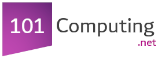Start

End
Create a program where the computer randomely seclects a number.
The program has to keep asking the user to guess the selected number until the user does.
import random
number = random.randint(1,10)
userGuess = int(input("Guess the number?"))
while userGuess != number:
userGuess = int(input("Try again. Type a number:"))
print("Well done, you guessed it right!")